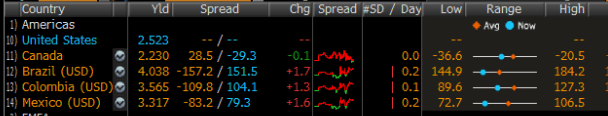# R shiny table with plots inside the table

I'm developing an R shiny app and ideally I would need to do precisely what is done here:More specifically, I have dataframe with stocks open, close, high, low data and I would need to replicate what's displayed in the attached image in column "Range". I understand I should attach some code, but the truth here, I can't find anything close to what I'm asking online. A sample dataframe would be:

``````df = data.frame(STOCK=c("IBM","MSFT","FB"),  OPEN=c(100,90, 80), CLOSE=c(102, 85, 82), LOW=c(99,81,78), HIGH=c(105, 91, 88))
``````

Then, I have no idea of what to do from here. Any suggestions? Thanks